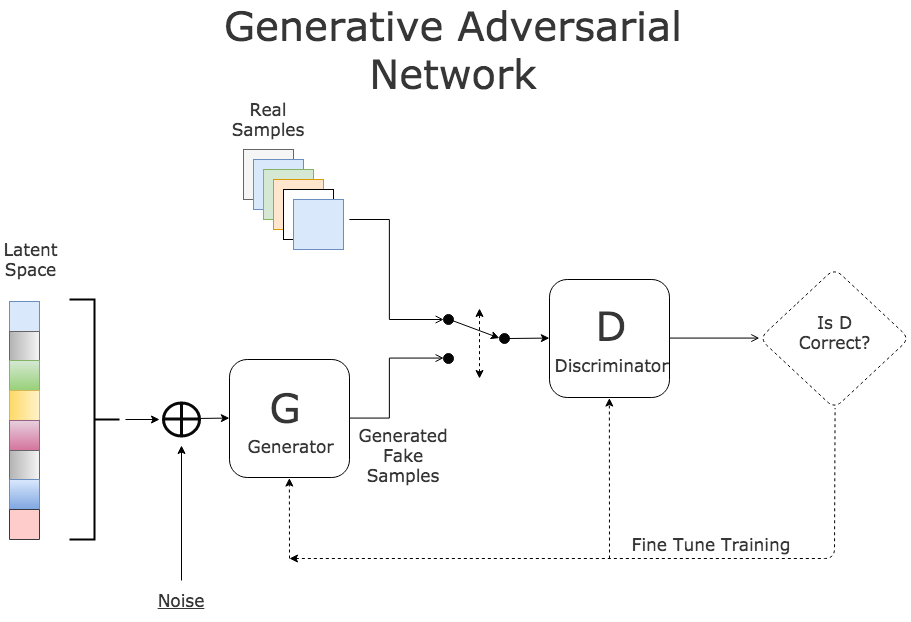# Overview: Generative Adversarial Networks – When Deep Learning Meets Game Theory

Before going into the main topic of this article, which is about a new neural network model architecture called Generative Adversarial Networks (GANs), we need to illustrate some definitions and models in Machine Learning and Artificial Intelligence in general.

Discriminative Models: Models that predict a hidden observation (called class) given some evidence (called features). In other words, we have some features and observations about an entity, and we want to predict its class, category or label. You can imagine the model as a function that has features as input and produces an output. The criteria that is used to produce the output depends on the model architecture and nature.

So, the discriminative model could be described in mathematical formula by f(x1, x2, x3, …, xn) = y, where n is the number of features, and the target of the function is to get the conditional probability P(y|x1, x2, x3, …, xn).

Support Vector Machines (SVMs) and Feedforward Neural Networks (FNNs) are examples of discriminative models that are used for classification.

Generative Models: Given some features, the models target is to learn how these features are produced, it tries to learn the distribution of the features. Assume that we have features x1, x2, x3, …, xn where n is the number of features, the model targets to learn the joint probability distribution of the features with the classes.

We can formulate this in mathematics by the joint probability P(x1, x2, x3, …, xn, y). After learning this distribution, we can estimate the conditional probability of the discriminative models to get the probability of the class given these features with their distribution.

Restricted Boltzmann Machines (RBMs) and Hidden Markov Models (HMMs) are examples of generative models. Note that, Vanilla Auto-encoders (AEs) aren’t considered to be a generative model, all what they do is just reconstruction of the features, on the other hand, Variational Auto-encoders (VAEs) belongs to generative models family.

Nash Equilibrium: A conceptual term that is used in Game Theory to describe a game situation in which the game players are satisfied by the decision he/she makes after revealing the other players strategies, and each player has no intention to change the strategy after knowing the other strategies as they didn’t effect on the strategy he/she used to win the game.

For example, assume we have a game in which each player has 2 choices to choose between them, and the 2 choices are known that are right and have the same effect regarding to the game points or rewards. The first player strategy maybe to choose the first choice, while the other player’s choice is the second one. After revealing that, each player is satisfied by the strategy he/she took because the other’s choice hasn’t effect badly to him/her.

Minimax: An algorithm that belongs to Game Theory and Statistics. The algorithm is used in games in which the game participants are 2 players, and each player tries to win the game by minimizing the worst case that is provided by the other player move, in other words, the player Minimize the Maximum move of the other player.

You can imagine the game of Chess, in which each player tries to win by making the best available move while the other player tries to minimize this move which is considered to be the best move by his side. Minimax is commonly used when making an AI-bot agent in Chess, Tic-tak-toc and Connect-4 games, you can generalize in the decision-making rule-based games.GANs consists of 2 models, a discriminative model (D) and a generative model (G). These models are participants on the training phase which looks like a game between them, and each model tries to better than the other.

The target of the generative model is to generate samples that are considered to be fake and are supposed to have the same distribution of the original data samples, on the other hand, the discriminative’s target is to enhance itself to be able to recognize the real samples among the fake samples generated by the generative model.

It looks like a game, in which each player (model) tries to be better than the other, the generative model tries to generate samples that deceives and tricks the discriminative model, while the discriminative model tries to get better in recognizing the real data and avoid the fake samples. It is as mentioned before, it is the same idea of the Minimax algorithm, in which each player targets to fail the other and minimize the supposed loss.

This game continues till we get a state, in which each model becomes an expert on what it is doing, the generative model increases its ability to get the actual data distribution and produces data like it, and the discriminative becomes expert in identifying the real samples, which increases the system’s classification task. In such case, we know that it reached that in which each model satisfied by its output (strategy), which is called Nash Equilibrium in Game Theory.

During the training phase, the loss function, that calculates the error, is used to update the 2 models parameters (learning the weights), also, the model can’t change the other’s parameters, the parameters are locally updated in each model using the global error.

This was an overview of this new arising model. I am still learning it and looking forward to using it in many applications, specifically in Natural Language Processing field.

References